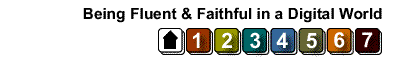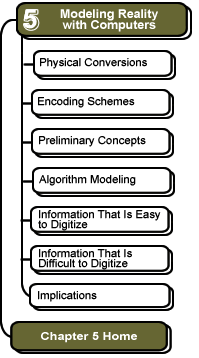# Preliminary Concepts: Negative Binary Numbers

Negative Numbers

We can represent negative numbers in several ways. The simplest is to simply use the leftmost digit of the number as a special value to represent the sign of the number: 0 = positive, 1 = negative. For example, a value of positive 12 (decimal) would be written as 01100 in binary, but negative 12 (decimal) would be written as 11100. Notice that in this system, it is important to show the leading 0 (to indicate a positive value).

For technical reasons, a different scheme, called "two's complement" is more often used for representing negative numbers. In this system, a positive 12 is still 01100, but -12 would be written as 10100. Notice that there is nothing instrinsically correct about one system over another. Either 11100 or 10100 can be used to represent -12, it just depends on what system of interpretation is used. That is, a human programmer chooses the meaning of the bits.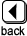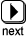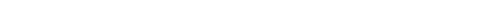These pages were written by Steven H. VanderLeest and Jeffrey Nyhoff and edited by Nancy Zylstra